# DAV Class 3 Maths Chapter 3 Worksheet 1 Solutions

The DAV Class 3 Maths Book Solutions and DAV Class 3 Maths Chapter 3 Worksheet 1 Solutions of Subtraction offer comprehensive answers to textbook questions.

## DAV Class 3 Maths Ch 3 WS 1 Solutions

Subtraction the following:

Question 1.Solution:
5237

Question 2.Solution:
3641

Question 3.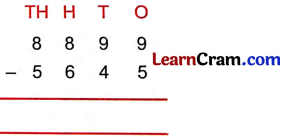Solution:
3254Question 4.Solution:
6121

Question 5.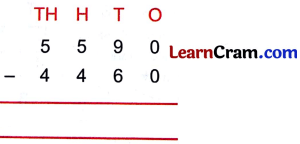Solution:
1130

Question 6.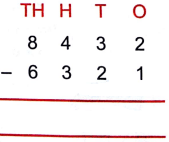Solution:
2111Question 7.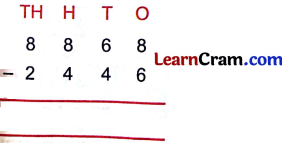Solution:
6422

Question 8.Solution:
526

Question 9.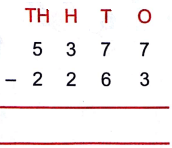Solution:
3114### DAV Class 3 Maths Chapter 3 Worksheet 1 Notes

Number – 0 = Number
Number – same number = 0

Subtraction of 10s, 100s, and 1000s
20 – 10 = 10
30 – 10 = 20
50 – 10 = 40
70 – 10 = 60
200 – 100 = 100
300 – 100 = 200
500 – 100 = 400
700 – 100 = 600
2000 – 1000 = 1000
3000 – 1000 = 2000
5000 – 1000 = 4000
7000 – 1000 = 6000
6000 – 1000 = 5000
7000 – 1000 = 6000
8000 – 1000 = 7000Question 1.
Collection of stamps of Rohan, Mohit, Renu and Neha are given below:Mohit = 359
Rohan = 682
Renu = 295
Neha = 800

(a) Who has the maximum number of stamps?
Solution:
Neha

(b) Who has the minimum number of stamps?
Solution:
Renu

(c) flow many more stamps does Neha have than Mohit?
Solution:
800 – 359 = 441

(cl) How much more is the collection of Rohan than Renu?
Solution:
682 – 295 = 387

Question 2.
A train was carrying 838 people. At one station 497 people got down from the train. How many people are left in the train?
Solution:
No. of people in the train = 838
No. of people got down = 497No. of people left in the train = 341.Question 3.
List of pocket money and money spent by three children are given below. Find out how much money is left with each of them?Solution:

 Name Pocket money Money spent Money left Akshay ₹ 275 ₹ 192 ₹ 83 Vineet ₹ 368 ₹ 259 ₹ 109 Sonal ₹ 700 ₹ 564 ₹ 136

Subtraction of 4-Digit Numbers

First subtract ones, next tens, then hundreds and lastly thousands.

Example: